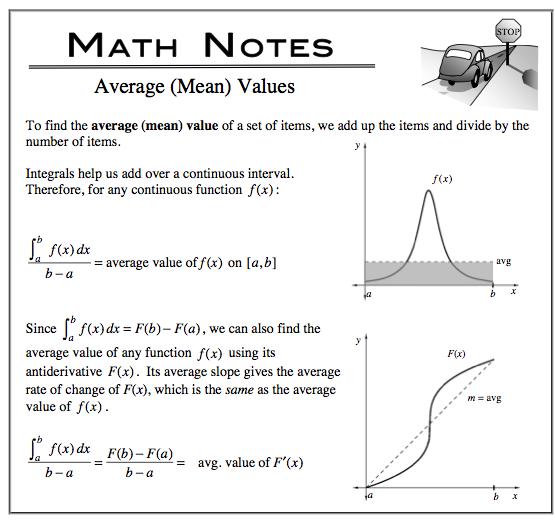### Home > CALC > Chapter 8 > Lesson 8.2.2 > Problem8-83

8-83.

Multiple Choice: The average value of the function $f (x) =x \sqrt { 1 - x ^ { 2 } }$ over the interval $0 ≤ x ≤ 1$ is:

1. $\frac { 1 } { 3 }$

1. $\frac { 1 } { 6 }$

1. $\frac { 2 } { 3 }$

1. $\frac { 1 } { 2 }$

1. $\frac { 1 } { 4 }$

Think about the information you are given. And think about what you want to know. Mean value of WHAT?
Will you integrate and then divide? Or will you compute the slope of the secant line?